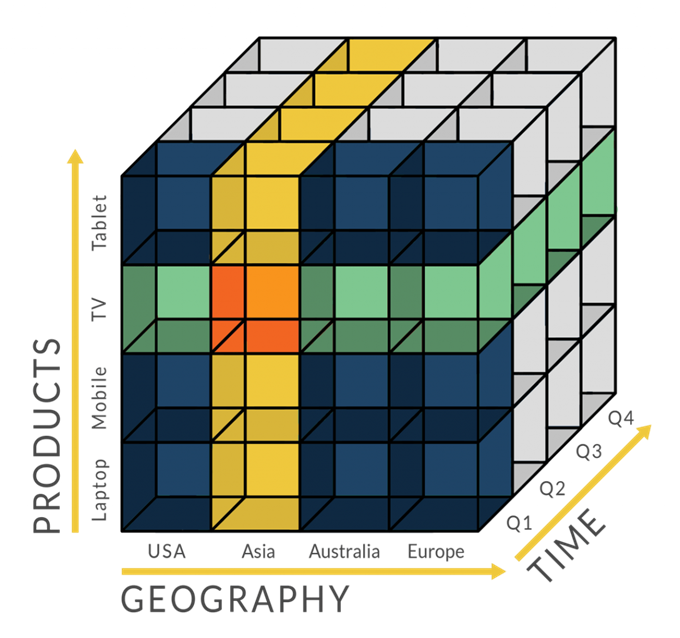# 游戏排名算法：Elo、Glicko、TrueSkill

## Elo等级分制度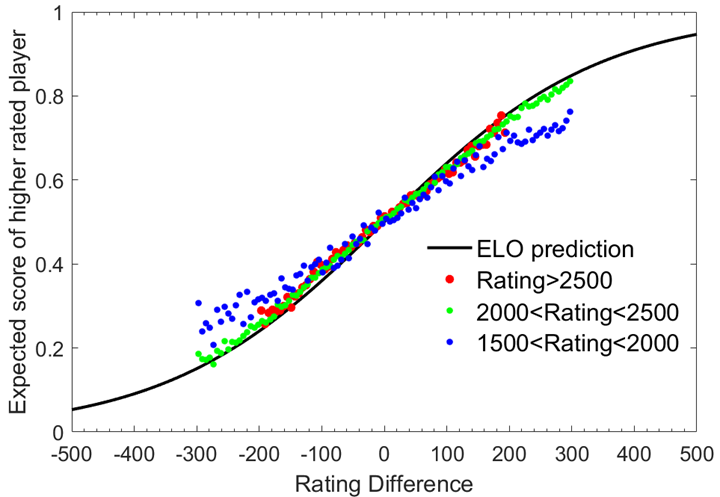ELO等级分制度是基于统计学的一个评估棋手水平的方法。美国国际象棋协会在1960年首先使用这种计分方法。由于它比先前的方法更公平客观，这种方法很快流行开来。1970年国际棋联正式开始使用等级分制度。Elo模型原先采用正态分布。但是实践显明棋手的表现并非呈正态分布，所以现在的等级分计分系统通常使用的是逻辑分布。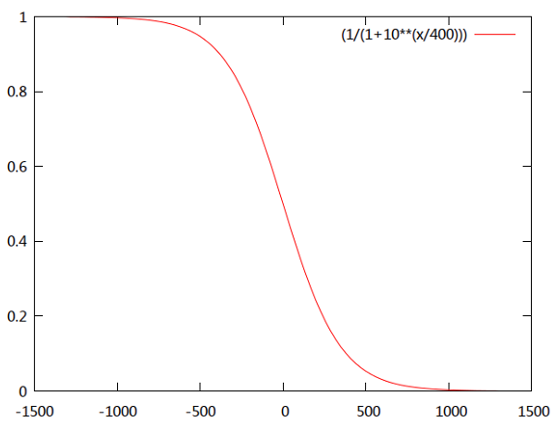$$E_{A}=\frac {1}{1+10^{(R_{B}-R_{A})/400}}$$

$$E_{B}=\frac {1}{1+10^{(R_{A}-R_{B})/400}}$$

$$R_{A}^{\prime }=R_{A}+K(S_{A}-E_{A})$$

### 国际足球等级分排名

Elo评级背后的基本原则是将所有球队以一个联赛的形式进行赛后积分统计，球队不比赛等级分不会变动，这与国际足联计算世界排名的方式并不相同。Elo自己有一个复杂全面且有效的权重评分系统，这与国际足联计分系统中的第一步也不相同。另外在国际足联系统中，球队会在赛后计算后直接获得现有分数，而Elo系统中只是算出加减分数，然后再得出当前分数。

$$R_{n}=R_{o}+KG(W-W_{e})$$

$$P=KG(W-W_{e})$$

• $R_{n}$ = 球队的新等级分
• $R_o$ = 球队的旧等级分
• K = 比赛的重要程度
• G = 净胜球数
• W =比赛结果
• $W_{e}$ = 预期结果
• P = 分数的变化

 比赛类型 指数 （K） 世界杯 60 洲际锦标赛和洲际锦标赛决赛 50 世界杯和洲际锦标赛的预选赛 40 其他所有比赛 30 友谊赛 20

 净胜球 K (G)系数 0 1 +1 1 +2 1.5 +3 1.75 +4 1.875 +5 2 +6 2.125 +7 2.25 +8 2.375 +9 2.5 +10 2.625

W是比赛的结果（胜一局为1，平一局为0.5，负一局为0）。注意：如果比赛拖进加时赛决胜负，那么加时赛的结果也会被计算在内。如果比赛由点球大战决定胜负，则比赛将会被判定为平局（W=0.5）。

$W_e$是比赛的预期结果，从下列公式得出：（比赛的结果符合预期为0.5）：

$$W_{e}=\frac {1}{10^{-dr/400}+1}$$

### 国际足联女子世界排名

$$R_{aft}=R_{bef}+K(S_{act}-S_{exp})$$

$$S_{exp}=\frac {1}{1+10^{-x/2}}$$

$$x=\frac {R_{bef}-O_{bef}\pm H}{c}$$

 进球差 0 1 2 3 4 5 6 /+ 非胜队的进球数 给予比例(百分比) 0 47 15 8 4 3 2 1 1 50 16 8.9 4.8 3.7 2.6 1.5 2 51 17 9.8 5.6 4.4 3.2 2 3 52 18 10.7 6.4 5.1 3.8 2.5 4 52.5 19 11.6 7.2 5.8 4.4 3 5 53 20 12.5 8 6.5 5 3.5

 进球差 1 2 3 4 5 6 /+ 落败队伍的进球数 给予比例(百分比) 0 85 92 96 97 98 99 1 84 91.1 95.2 96.3 97.4 98.5 2 83 90.2 94.4 95.6 96.8 98 3 82 89.3 93.6 94.9 96.2 97.5 4 81 88.4 92.8 94.2 95.6 97 5 80 87.5 92 93.5 95 96.5

 重要比赛 重要比赛系数(M) K-value 国际足联女子世界杯比赛 4 60 女子奥运足球比赛 4 60 女子世界杯外围赛 3 45 女子奥运会外围赛 3 45 女子洲区决赛圈 3 45 女子洲区外围赛 2 30 两队间前10友谊赛 2 30 友谊赛 1 15

### 国际足联世界排名

2018年6月10日，国际足联公布了最新的排名系统：

$$P=P_{\text{before}}+I(W-W_{e})$$

• $P_{before}$ – 球队比赛前的积分
• I – 赛事重要性系数：
• 05 – 于国际赛期期间以外的友谊赛
• 10 – 于国际赛期期间之内的友谊赛
• 15 – 国家联赛赛事（小组赛）
• 25 – 国家联赛赛事（附加战平决赛）
• 25 – 洲际赛预选赛赛事、世界杯预选赛
• 35 – 洲际赛决赛圈赛事（四分之一决赛阶段以前）
• 40 – 洲际赛决赛圈赛事（四分之一决赛阶段以后）
• 50 – 国际足联世界杯赛事（四分之一决赛阶段以前）
• 60 – 国际足联世界杯赛事（四分之一决赛阶段以后）
• W – 比赛成绩：(假如一场赛事最终分出胜负，但仍然需要进行点球大战（意即两回合中的次回合赛事），这会被视为一场常规赛的胜负，而点球大战成绩不会计算。)
• 0 – 于常规时间或加时赛后落败
• 5 – 战平或于点球大战落败
• 75 – 于点球大战胜出
• 1 – 于常规时间或加时赛后胜出
• We – 赛事之预计赛果：$W_{e}=\frac {1}{10^{-{\frac {dr}{600}}}+1}$,其中 dr 是两支球队之间比赛前的评分差

## Glicko评分系统

Glicko评分系统（英文：Glicko rating system）及Glicko-2评分系统（英文：Glicko-2 rating system）是评估选手在比赛中（如国际象棋及围棋）的技术能力方法之一。此方法由马克·格利克曼发明，原为国际象棋评分系统打造，后作为等级分评分系统的改进版本广泛应用。格里克曼在此算法中的主要贡献是“评分可靠性”（Ratings Reliability，简称RD），即评分标准差（Ratings Deviation）。

Glicko与Glicko-2评分系统被发表至公有领域。诸多在线游戏服务器（如《Pokémon Showdown》、《Lichess》、《自由互联网国际象棋服务器》、《Chess.com》、《在线围棋服务器 （页面存档备份，存于互联网档案馆）》、《反恐精英：全球攻势》、《军团要塞2》、《刀塔霸业》、《激战2》、《Splatoon 2》及《皇舆争霸》）和多个竞技性编程比赛都采用此种评分方法。Glicko所使用的算法可在其网站上找到。

Glicko-2是Glicko评分系统的改进版本，引进了评分挥发度$\sigma$（Rating Volatility）的概念。澳大利亚国际象棋联盟采用稍加修改版的Glicko-2评分系统。

### Glicko

Elo系统的问题在于无法确定选手评分的可信度，而Glicko系统正是针对此进行改进。假设两名评分均为1700的选手A、B在进行一场对战后A获得胜利，在美国国际象棋联赛的Elo系统下，A选手评分将增长16，对应地B选手评分将下降16。但是加入A选手是已经很久没玩，但B选手每周都会玩，那么在上述情况下A选手的1700评分并不能十分可信地用于评定其实力，而B选手的1700评分则更为可信。

$$RD=\min ({\sqrt {{RD_{0}}^{2}+c^{2}t}},350)$$

t为自上次比赛至现在的时间长度（评分期），350则是新选手的评分标准差。若选手在一个评分期间内进行了多场比赛，此算法会将进行的比赛作为一场看待。评分期根据选手进行比赛的频繁程度，可能长至七个月，短至几分钟。常数c根据选手在特定时间段内的技术不确定性计算而来，计算方法可能通过数据分析，或是估算选手的评分标准差将在什么时候达到未评分选手的评分标准差得来。若一名选手的评分标准差将在100个评分期间内达到350的不确定度，则评分标准差为50的玩家的常数c可通过解$350=\sqrt {50^{2}+100c^{2}}$的方式计算而来。或$c=\sqrt {(350^{2}-50^{2})/100}\approx 34.6$

$$r=r_{0}+{\frac {q}{{\frac {1}{RD^{2}}}+{\frac {1}{d^{2}}}}}\sum _{i=1}^{m}{g(RD_{i})(s_{i}-E(s|r_{0},r_{i},RD_{i}))}$$

• $g(RD_{i})=\frac {1}{\sqrt {1+{\frac {3q^{2}(RD_{i}^{2})}{\pi ^{2}}}}}$
• $E(s|r,r_{i},RD_{i})=\frac {1}{1+10^{\left({\frac {g(RD_{i})(r_{0}-r_{i})}{-400}}\right)}}$
• $q=\frac {\ln(10)}{400}=0.00575646273$
• $d^{2}=\frac{1}{q^{2}\sum _{i=1}^{m}{(g(RD_{i}))^{2}E(s|r_{0},r_{i},RD_{i})(1-E(s|r_{0},r_{i},RD_{i}))}}$
• $r_{i}$表示选手个人的评分；
• $s_{i}$表示每场比赛后的结果。胜利为1，平局为$\frac {1}{2}$，失败为0。

$$RD’=\sqrt {({\frac {1}{RD^{2}}}+{\frac {1}{d^{2}}})^{-1}}$$

### Glicko-2

Glicko-2 系统中的每个玩家都有一个评分 r、一个评分偏差 RD 和一个评分波动率$\sigma$。波动性度量表明玩家评级的预期波动程度。当球员表现不稳定时（例如，当球员在稳定一段时间后取得了异常强劲的成绩）时，波动性衡量指标较高，而当球员表现稳定时，波动性衡量指标较低。与最初的 Glicko 系统一样，通常以间隔的形式总结球员的实力（而不仅仅是报告评级）。一种方法是报告 95% 的置信区间。区间中的最小值是玩家的评分减去两倍的RD，最高值是玩家的评分加上两倍的RD。因此，例如，如果某个玩家的评分为 1850，RD 为 50，则该区间将从 1750 到 1950。那么我们可以说我们有 95% 的把握认为该玩家的实际实力在 1750 到 1950 之间。当 a球员的 RD 较低，区间会很窄，因此我们有 95% 的信心认为球员的实力处于较小的数值区间。波动率度量没有出现在这个区间的计算中。

• 如果玩家未评级，将评级设置为 1500，将 RD 设置为 350。将玩家的波动率设置为06（该值取决于特定的应用程序）。
• 否则，使用玩家最近的评分、RD 和波动率$\sigma$

$$\mu = (r – 1500)/173.7178$$

$$\phi = RD/173.7178$$

$\sigma$的值，即波动率，不会改变。

$$v=[\sum_{j=1}^{m} g(\phi_{j})^{2} \mathrm{E}(\mu, \mu_{j}, \phi_{j})\{1-\mathrm{E}(\mu, \mu_{j}, \phi_{j})\}]^{-1}$$

$$g(\phi) =\frac{1}{\sqrt{1+3 \phi^{2} / \pi^{2}}}$$

$$\mathrm{E}(\mu, \mu_{j}, \phi_{j}) =\frac{1}{1+\exp (-g(\phi_{j})(\mu-\mu_{j}))}$$

$$\Delta=v \sum_{j=1}^{m} g(\phi_{j})\{s_{j}-\mathrm{E}(\mu, \mu_{j}, \phi_{j})\}$$

g()和 E()在上一个步骤已经定义过了。

1.让$a=\ln(\sigma^2)$，并定义

$$f(x)=\frac{e^{x}(\Delta^{2}-\phi^{2}-v-e^{x})}{2(\phi^{2}+v+e^{x})^{2}}-\frac{(x-a)}{\tau^{2}}$$

2.设置迭代算法的初始值。

1. 让k=1
2. 如果$f(a-k\tau )<0$，然后设置k=k+1，然后继续执行(2)

3.让$f_A = f(A)$和$f_B = f(B)$

4.当$|B-A|>\varepsilon$，执行以下步骤。

• (a)让$C=A+(A-B)f_A/(f_B-f_A)$，让$f_C=f(C)$。
• (b)如果$f_Cf_B<0$，然后设置$A \leftarrow B$和$f_A \leftarrow f_B$；否则，只需设置$f_A \leftarrow f_A/2$。
• (c)设置$B \leftarrow C$ 和$f_B \leftarrow f_C$
• (d)如果$|B-A| \leq \varepsilon$停止，否则重复以上三步。

5.一旦$|B-A| \leq \varepsilon$，设置$\sigma ^{‘} \leftarrow e^{A/2}$

$$\phi ^* = \sqrt{\phi ^2+\sigma ‘^{2}}$$

$$\phi^{\prime} =1 / \sqrt{\frac{1}{\phi^{* 2}}+\frac{1}{v}}$$

$$\mu^{\prime} =\mu+\phi^{\prime 2} \sum_{j=1}^{m} g(\phi_{j})\{s_{j}-\mathrm{E}(\mu, \mu_{j}, \phi_{j})\}$$

$$r’=173.7178u’+1500$$

$$RD’=173.7178\phi ‘$$

$$\phi^{\prime}=\phi^{*}=\sqrt{\phi^{2}+\sigma^{2}}$$

## TrueSkill

• 一个包含跟踪所有玩家比赛结果，记录玩家能力的模块。
• 一个对比赛成员进行配对的模块。
• 一个公布比赛中各成员能力的模块。

• 适用于复杂的组队形式，更具一般性。
• 置于更完善的建模体系，容易扩展。
• 继承了贝叶斯建模的好的特点，如模型选择等。

TrueSkill排名系统是针对玩家能力进行设计的，以克服现有排名系统的局限性，确保比赛双方的公平性，可以在联赛中作为排名系统使用。它为玩家排名使用的为贝叶斯定理。 系统的特点是假设每一个玩家的能力不是固定的，其能力水平的表现为一个钟型曲线（正态分布或高斯分布）。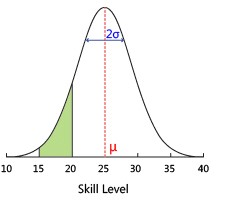TrueSkill排名系统只会根据比赛结果更新$\mu$和$\sigma$，它假设的情况为一个玩家的表现围绕着他的能力水品进行变化，如果一个玩家玩一个基于点数的游戏，他战胜了所有的其他10个对手和他和战胜了另外一场比赛只有一个对手的积分是一样的，但是这样两场比赛确实反映了不同选手的能力情况。通常会使$\sigma$下降。在计算一场新的比赛结果之前，TrueSkill排名系统会计算比赛的排名与选手在比赛前的排名的变化情况。排名的变化最终影响了玩家技能的不确定性$\sigma$。这个参数可以被TrueSkill用来记录玩家的技能的变化。并且$\sigma$永远不可能为0。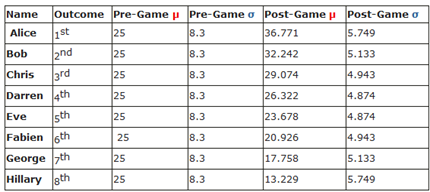\begin{aligned}&\mu_{winner} \longleftarrow \mu_{winner}+\frac{\sigma_{winner}^{2}}{c} * v(\frac{\mu_{winner}-\mu_{loser }}{c}, \frac{\varepsilon}{c}) \\&\mu_{loser} \longleftarrow \mu_ {loser}-\frac{\sigma_{loser}^{2}}{c} * v(\frac{\mu_{winner}-\mu_{loser}}{c}, \frac{\varepsilon}{c}) \\&\sigma_{winner}^{2} \longleftarrow \sigma_{winner}^{2} *[1-\frac{\sigma_{winner}^{2}}{c} * w(\frac{\mu_{vinner}-\mu_{loser}}{c}, \frac{\varepsilon}{c}). \\&\sigma_{loser}^{2}<\sigma_{loser}^{2} * [1-\frac{\sigma_{loser}^{2}}{c} * w(\frac{\mu_{winner}-\mu_{loser}}{c}, \frac{\varepsilon}{c}). \\&c^{2}=2 \beta^{2}+\sigma_{winner}^{2}+\sigma_{loser}^{2}\end{aligned}

$$e^{-\frac{(\mu_{A}-\mu_{B})^{2}}{2 c^{2}}} \sqrt{\frac{2 \beta^{2}}{c^{2}}}$$

c的值由如下公式给出

$$c^{2}=2 \beta^{2}+\mu_{A}^{2}+\mu_{B}^{2}$$

TrueSkill假设玩家的水平可以用一个正态分布来表示，而正态分布可以用两个参数：平均值和方差来完全描述。设Rank值为R，代表玩家水平的正态分布的两个参数平均值和方差分别为$\mu$和$\sigma$，则系统对玩家的评分即Rank值为 R=$\mu$-k*$\sigma$。k值越大则系统的评分越保守。在Xbox Live上，系统为每个玩家赋予的初值是$\mu = 25$以及 $\sigma = 25/3$，k=3。所以玩家的起始Rank值为R=25-3*25/3=0。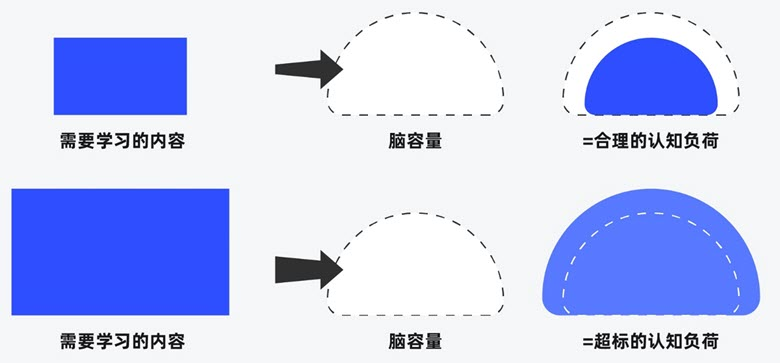##### 界面设计和游戏设计中的认知负荷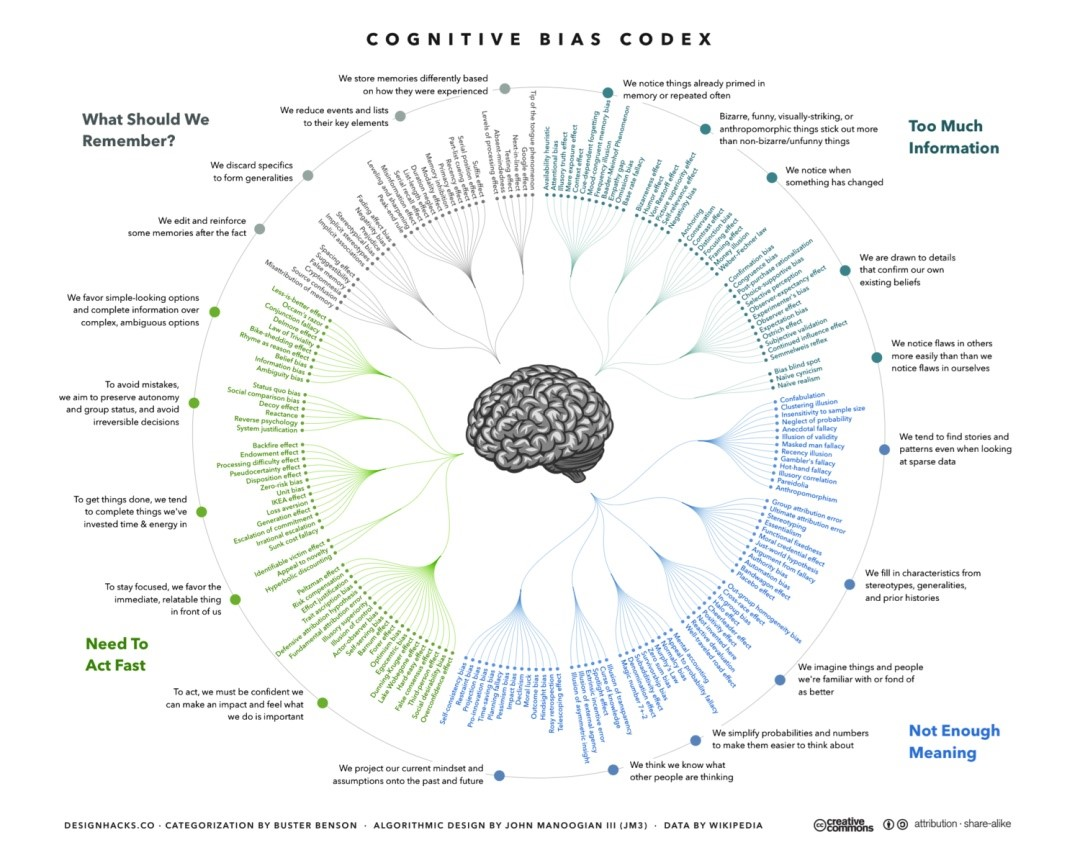##### 理解认知偏差